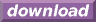#Calcute: freeware calculator

## Inverse (inv)

The multiplicative inverse of a number is the value which, when multiplied by the original number, produces 1. Calcute implements it using the inv function. For a real number x, inv(x) = 1/x. For a ratio a/b, inv(a/b) = b/a. The function is undefined at zero.

```inv(2)
0.5
```

The term "reciprocal" has also been used to denote the multiplicative inverse. The term "inverse" can also be used for other functions that are not related to multiplication, at which time the word is qualified by an adjective. Common usage of "inverse" without any qualifier refers to the multiplicative inverse specifically.